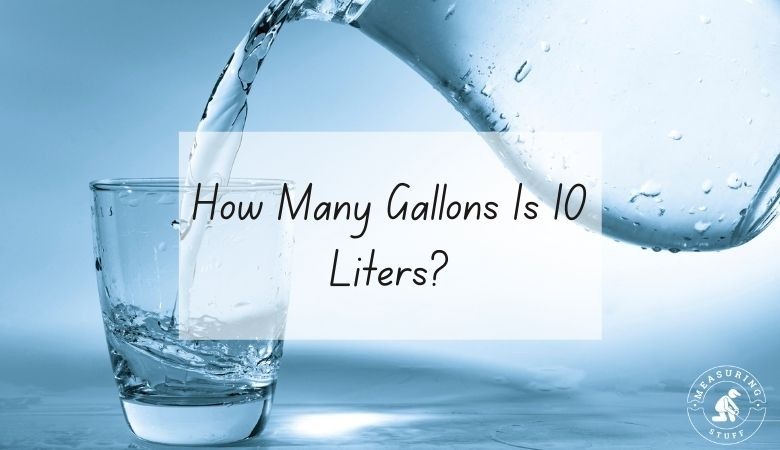# How Many Gallons Is 10 Liters?

This article will show you how many gallons are in 10 liters.

Liters and gallons are similar units of measurement in that they’re both designed to measure volume.

There is a simple formula that can help you quickly figure out how many liters are in a gallon.

There are about .26 gallons to every one liter.

To find out how many liters are in a gallon, simply divide the number of liters by 3.785.

Liters / 3.785 = Gallons.

For example, if you have 10 liters, divide it by 3.785 to get 2.642 which is the approximate number of gallons in 10 liters.

If you want to find out how many gallons there are in a certain number of liters, you can reverse the equation.

Instead, multiply the number of gallons by 3.785.

Gallons x 3.75 = Liters.

For example, if you have 10 gallons, multiply that by 3.785 to get 37.85, which is the number of liters in 10 gallons.

If you don’t feel like doing the math, you can use our online conversion calculator below.

## Liters To Gallons Conversion Calculator

Use the following calculator to easily convert liters into gallons. Simply type in the desired value and select liters in the convert from box and gallons in the convert to box. The calculator will instantly do the math for you.

Check out all our conversion calculators here.

Other articles on our site using liters and gallons include:

You can also convert 10 liters into other units of measurements.

10 liters = 41.666 cups

10 liters = 10.566 quarts

10 liters = 10000 milliliters

## Summary

You now know how many gallons are in 10 liters and you should be able to convert 10 liters into gallons easily. Feel free to share our page on social media and save our website in your bookmarks for easy and quick reference.